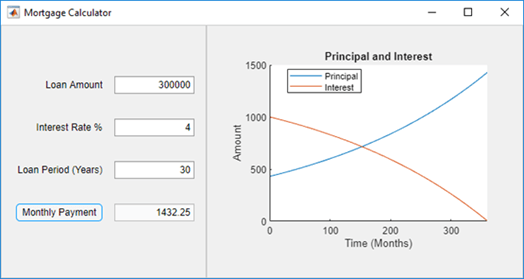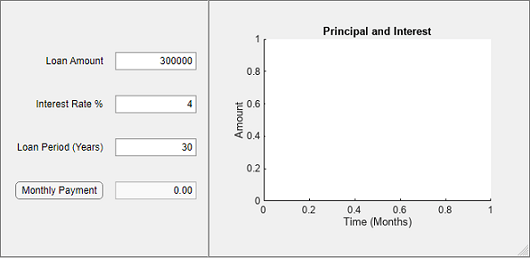# Plot Numeric Data in an App

In App Designer, you can create an app that plots numeric data and updates in response to user inputs. The app in this example collects user input, calculates monthly payments based on the input, and then plots the principal and interest amounts over time. This example demonstrates these app building tasks:

• Access property values from UI components.

• Plot data within an app.### Lay Out UI Components in Design View

This app uses these components to create the simple mortgage amortization calculator:

• Numeric edit fields — Enter numeric values, such as the loan amount, interest rate, and loan period, and view the calculated monthly payment amount. MATLAB® automatically checks to make sure the values are numeric and within the range you specified.

• Push button — Calculate the monthly payment value from the provided inputs.

• Axes — Display a plot of principal and interest amounts verses mortgage installment.

Lay Out UI Components

To create the mortgage calculator, start by opening a new two-panel app with auto-reflow from the App Designer Start Page. In Design View, drag numeric edit fields, a push button, and axes from the Component Library onto the app canvas.

Configure Component Appearance

You can modify the appearance of a component by editing the component directly on the canvas or by changing properties in the Component Browser. For example:

• Edit the label of a component interactively. Double-click the edit field labels on the app canvas and modify the label text.

• Turn off the ability to edit data in the Monthly Payment edit field. Select the edit field component and then clear the Editable check box in the Component Browser.

After laying out the app components, the app canvas in Design View shows fields for the app user to input the loan amount, interest rate, and loan period, as well as a button to calculate the monthly payment, which does not have an effect yet. The Principal and Interest plot is empty. For more information about arranging app components, see Lay Out Apps in App Designer Design View.### Program App Behavior in Code View

To program the app behavior in Code View, use callback functions that execute whenever the app user interacts with specific app components. For example, write a callback function for the Monthly Payment button to calculate the monthly payment and plot some data when the user pushes the button.

Add a `ButtonPushedFcn` callback function for the Monthly Payment button by right-clicking `app.MonthlyPaymentButton` in the Component Browser. Then select Callbacks > Add ButtonPushedFcn callback in the context menu. App Designer automatically generates a blank function in Code View and assigns it as a callback function to the button. For more information about callbacks, see Callbacks in App Designer.

Then within the callback function you just created, add code to calculate the monthly payment and plot the principal and interest amounts over time. Because the callback is assigned to the button, when a user clicks the Monthly Payment button, this code executes.

Calculate the Monthly Payment

Access the numeric input values of your app components by using the dot notation `app.ComponentName.Property`. For example, store the input from the Loan Amount field into a local variable within the callback function.

```amount = app.LoanAmountEditField.Value; ```

Using the inputs from the numeric edit fields for Loan Amount, Interest Rate, and Loan Period, calculate the monthly payment within the callback function.

```payment = (amount*rate)/(1-(1+rate)^-nper); ```

To output the calculated payment amount in the Monthly Payment numeric edit field, set the Monthly Payment value to the calculated payment amount.

```app.MonthlyPaymentEditField.Value = payment; ```

Plot the Data in the Axes

To generate the principal and interest amounts from the Monthly Payment value, start by preallocating and initializing variables.

```interest = zeros(1,nper); principal = zeros(1,nper); balance = zeros(1,nper); balance(1) = amount; ```

Then calculate the principal and interest.

```for i = 1:nper interest(i) = balance(i)*rate; principal(i) = payment - interest(i); balance(i+1) = balance(i) - principal(i); end ```

Plot data within an app by using the `plot` function and specifying the `UIAxes` object in your app as the first argument. For example, plot the principal and interest amounts on the axes named `PrincipalInterestUIAxes`.

```plot(app.PrincipalInterestUIAxes,(1:nper)',principal,(1:nper)',interest); ```

Edit the Axes Appearance

Adjust the axis limits and labels by specifying the `UIAxes` object as the first argument in the corresponding functions. For example, add a legend to the axes and adjust the axes limits by specifying `app.PrincipalInterestUIAxes` as the first input argument in the `legend`, `xlim`, and `ylim` functions, respectively.

```legend(app.PrincipalInterestUIAxes,{"Principal","Interest"},"Location","Best"); xlim(app.PrincipalInterestUIAxes,[0 nper]); ylim(app.PrincipalInterestUIAxes,"auto"); ```

Run the App

To run the app, click Run in the App Designer toolstrip. Enter some values in the numeric fields and click the Monthly Payment button. The app calculates the monthly payment and plots the principal and interest data.